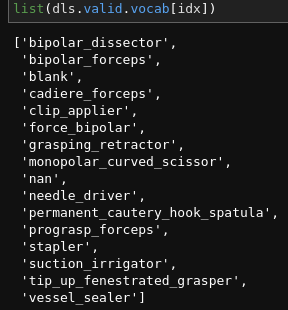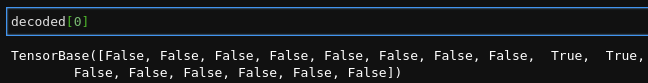# Confusion Matrix in MultiCategory() block is not showing all classes of the validation set

Hi,

I have the following specification for the datablock to train a multicategory computer vision model:

dblock = DataBlock(
blocks=(ImageBlock,MultiCategoryBlock()),
get_x=get_x,
get_y=get_y,
splitter=splitter,
item_tfms=Resize((90,160), method=ResizeMethod.Squish),
batch_tfms=[*aug_transforms(size=(90,160), min_scale=1),Normalize.from_stats(*imagenet_stats)])


I used it to train a convnext model which went fine but when I printout the confusion matrix for the learner, it is only showing two classes as follows:

I thought that the validation set may not contain all the classes so I run the following code to verify the class labels in the validation set:

dsets = dblock.datasets(images_df)
ys = [i for i in dsets.valid] # took a lot of time. There might be a bettter way to get ys from dls.valid
idx=[]
for item in ys:
for i,y in enumerate(item):
if y.item()==1.0:
idx.append(i)
idx=list(set(idx))
list(dls.valid.vocab[idx])


So I was wrong as the validation set contained all the class labels. See the output below:Did anyone else encounter the same issue? Any guidance in fixing it will be much appreciated.

Best regards,
Bilal

1 Like

Hey,
as far as I understand you are using multi label classification. By default the fastai learner uses BCECrossEntropyFlatten as a loss function in that case. The ClassificationInterpretation expects label predictions (so 0, 1, 2, 3,…) but the model predicts True/False (1/0) values per instance per label, that’s why you only see those two values in the confusion matrix. I don’t think that fastai offers a multi label confusion matrix out of the box…

1 Like

Hey benkarr,

Thanks for pointing out the issue. I’ve explored the code and found get_preds returns the list of booleans for each label per instance as you mentioned.The following code in confusion_matrix(self) method needs changing to compute the cm for class labels indexes 0,1,2,... not the True/False flattened lists.

cm = ((d==x[:,None]) & (t==x[:,None,None])).long().sum(2)

This is the tricky bit1 Like

I thought about making a change at that point too, but I’m not shure if that leads to something usefull…It’s hard to describe, but think about misclassification: say the true label of an instance is [A, B] but you classified it as [A, C]. How would you capture that in a 2D matrix? Would C be a misclassification for A or for B? But A was classified correctly! Do you get the weirdness?A quick search brought up sklearns multilabel_confusion_matrix which gives a 2 \times 2 [[TP,FP],[FN, TN]] matrix for every label, maybe that could be useful for interpreting the results. (It takes the n_instances\timesn_classes boolean arrays that you get from the get_preds.)

2 Likes

Thanks. I tried multilabel_confusion_matrix() for the targs and decoded returned from get_preds() method like below:

multilabel_confusion_matrix(targs, decoded)

The result is as you described:

array([[[201978,      0],
[     1,    122]],

[[ 94519,    645],
[   464, 106473]],

[[200914,      1],
[     0,   1186]],

[[ 87634,   2076],
[  1978, 110413]],

[[196506,    683],
[   616,   4296]],

[[189147,    194],
[   323,  12437]],

[[184899,    380],
[  1405,  15417]],

[[104251,    793],
[  1198,  95859]],

[[  1186,      0],
[     1, 200914]],

[[119364,    435],
[   328,  81974]],

[[194873,    204],
[   210,   6814]],

[[178674,    291],
[   438,  22698]],

[[200992,     48],
[   222,    839]],

[[201978,      0],
[    27,     96]],

[[201105,      4],
[    40,    952]],

[[193801,    294],
[   227,   7779]]])


These are confusion matrices for each class label but can’t provide insights such as how many times class A is classified as A or B and so on.

This seems to be a tough to achieveThere is an attempt to visualise a multi-label confusion matrix on stackoverflow. I’m not sure that it would work well as the number of labels increase, would quickly run out of screen space (scikit learn - Plot Confusion Matrix for multilabel Classifcation Python - Stack Overflow).

1 Like

What if we introduce a null label to capture the misclassification of labels that are not in the actual labels (targs) or the ones not predicted by the algorithm at all? In the same example, for the true label of an instance [A, B] and prediction as [A, C], we will say C be the misclassification for null and likewise B is misclassified as null. Only A was classified correctly.

Will it make sense?

Thanks AllenK, I will have a look into this.

Yes agreed! It is difficult to quantify the misclassification rate at an individual class level I think. There are a couple of things that come to my mind.

We can create a plain CM of counts of actual classes vs predicted classes. Now, this won’t hav the nice properties of row sum being equal to count of actual ground truth labels etc. but if two or more labels appear together and they’re all predicted correctly by the algorithm, then the resulting CM would always be symmetric or near symmetric. This way we can check the overall performance of our model.

Also, on a tangent, I find it useful to compute f1-score at a sample level aggregate to get an insight into how accurate on a sample level basis my trained model is!

Thanks,
Vinayak.

The more I think through the issue, the more it becomes clearer that the confusion matrix will mislead the conclusion. Bcz we can’t really say B is the misclassification of C. So, there is no point in creating the confusion matrix for the multicategory model.

Thanks to everyone for providing such a good explanation to understand the issue.

1 Like

Had an idea of one approach that may be useful is to generate 2 confusion matrices for multi-label cases.

1 for the Positives. to see where most of the False Positives occur.
1 for the Negatives. to see where most of the False Negatives occur

Thanks AllenK. This sounds like a good idea. Will give it a try.

2 Likes

In my opinion, all suggestions for capturing the results, that came up here go into the same direction as the sklearn method. I stiched together a notebook about multilabel_confusion_matrix, mainly to clarify it for myself, but maybe someone else finds that usefull too2 Likes